Geometry Vocab
Transversals
Parallelograms
100

What is this?A ray100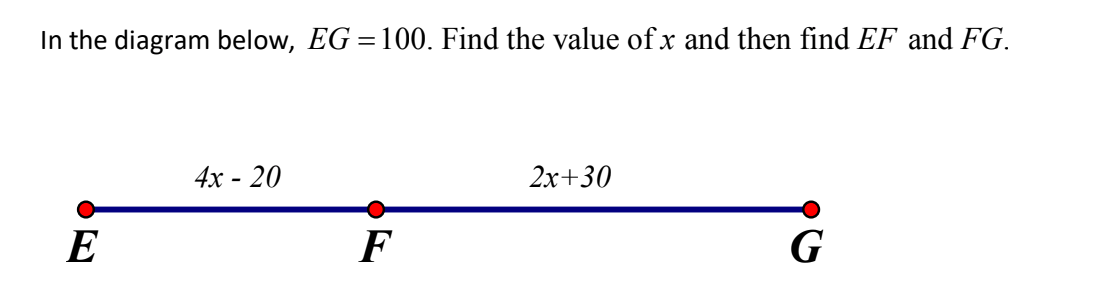x = 15
EF = 40
FG = 60

100

What are these angles called?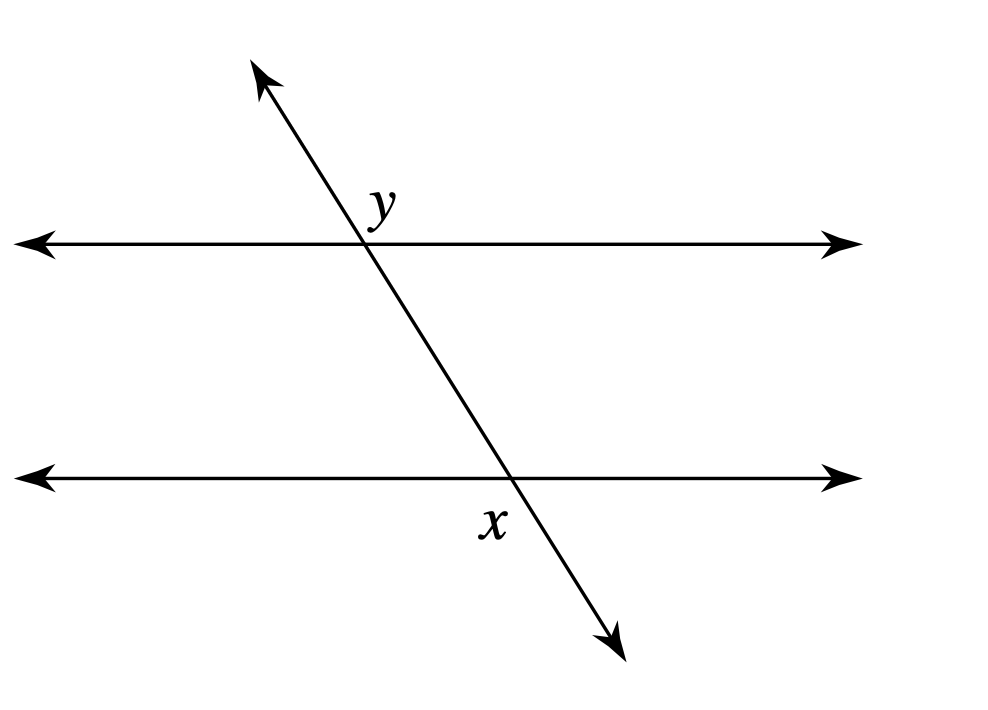Alternate Exterior

100

Find the measure of the unknown angle135

100

Where did Isaac go to college?

Seattle U and UW

200

Name the ray200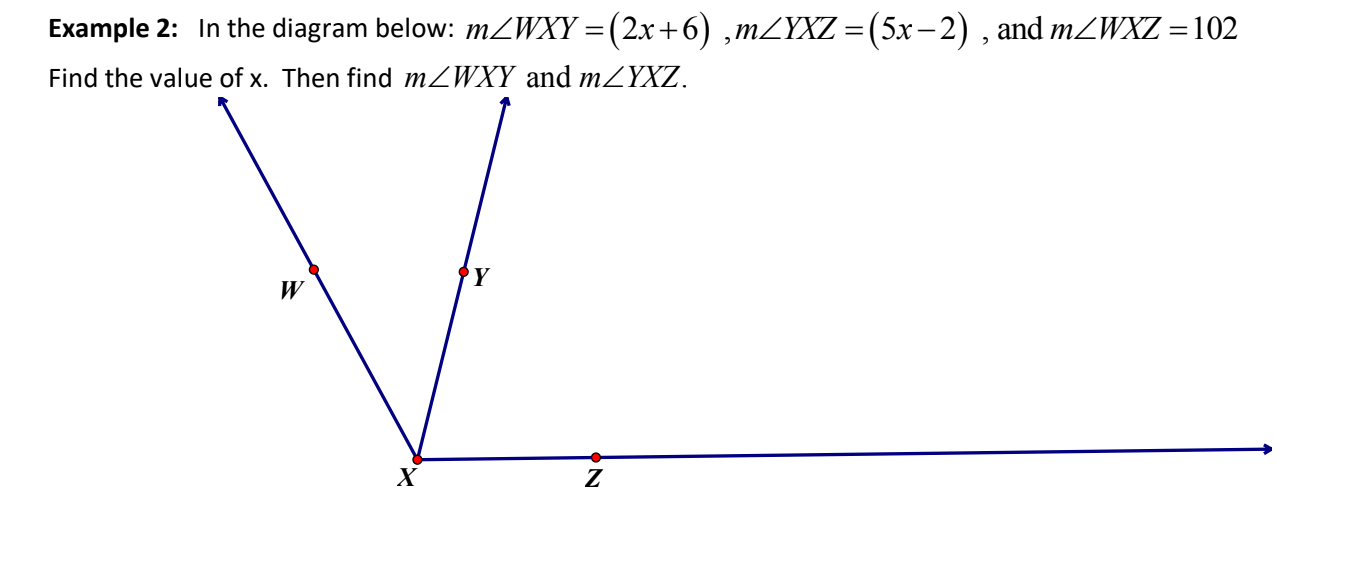x = 14

m\angle WXY = 34^o

m\angle YXZ = 68^o

200

Find the measure of the unknown angle111

200

Find the measure of the unknown angles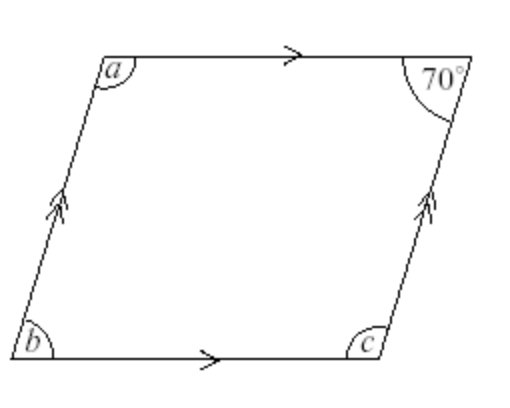a = 110

b = 70
c = 110

200

Where did Isaac grow up?

Spokane

300

What is the wrong way to name the angle?\angle ABC

300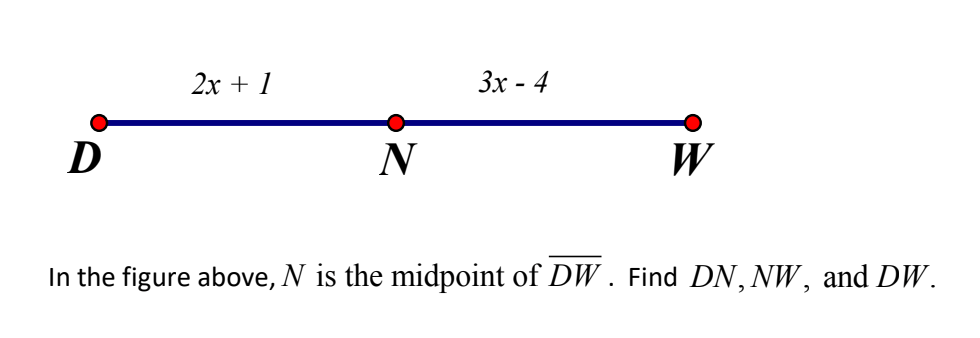DN = 11
NW = 11
DW = 22

300

What is the measure of the angle?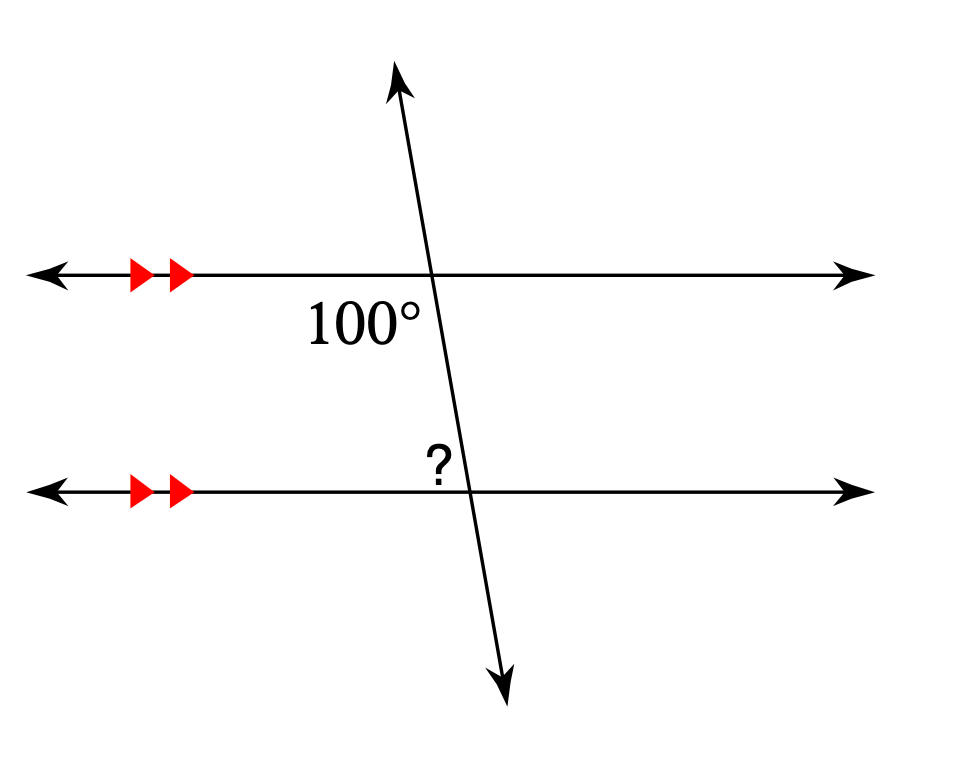80

300

Find the measure of the unknown angles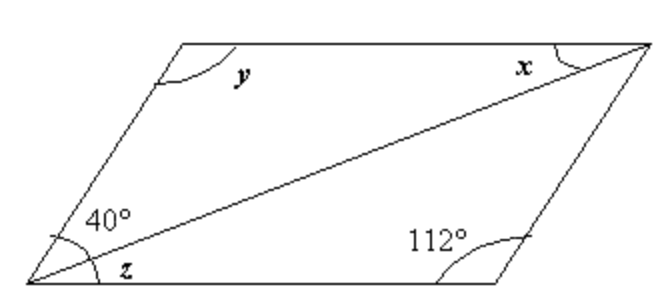x = 28
y = 112
z = 28

300

How many siblings does Isaac have?

Two younger sisters!
400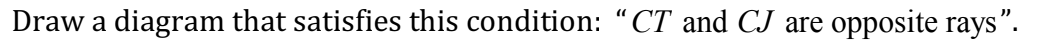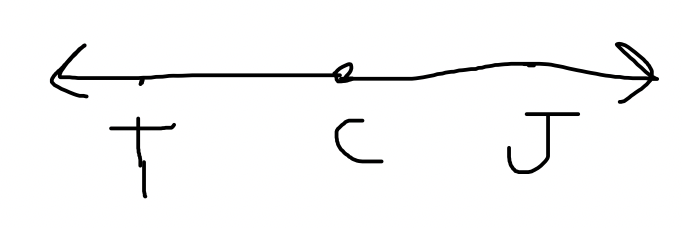400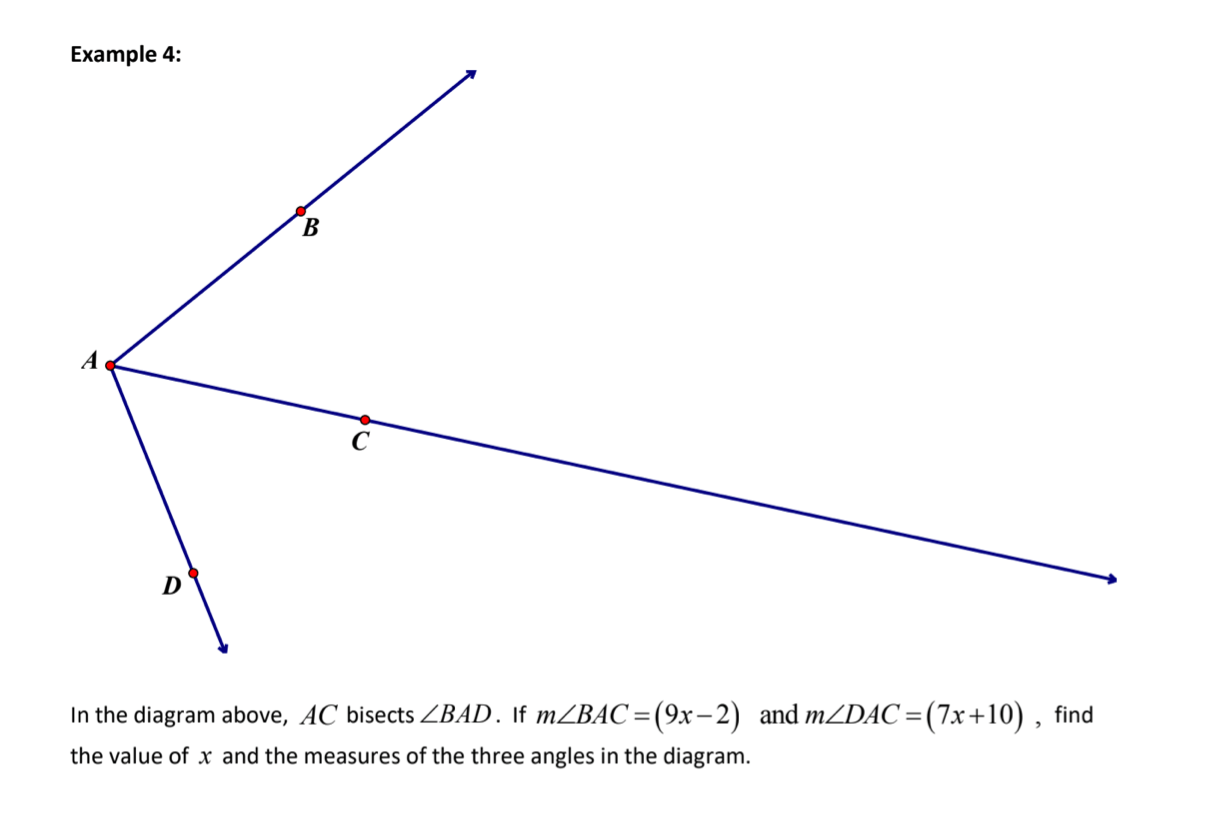x = 6

m\angle BAC = 52^o

m\angle DAC = 52^o

400

Find the value of x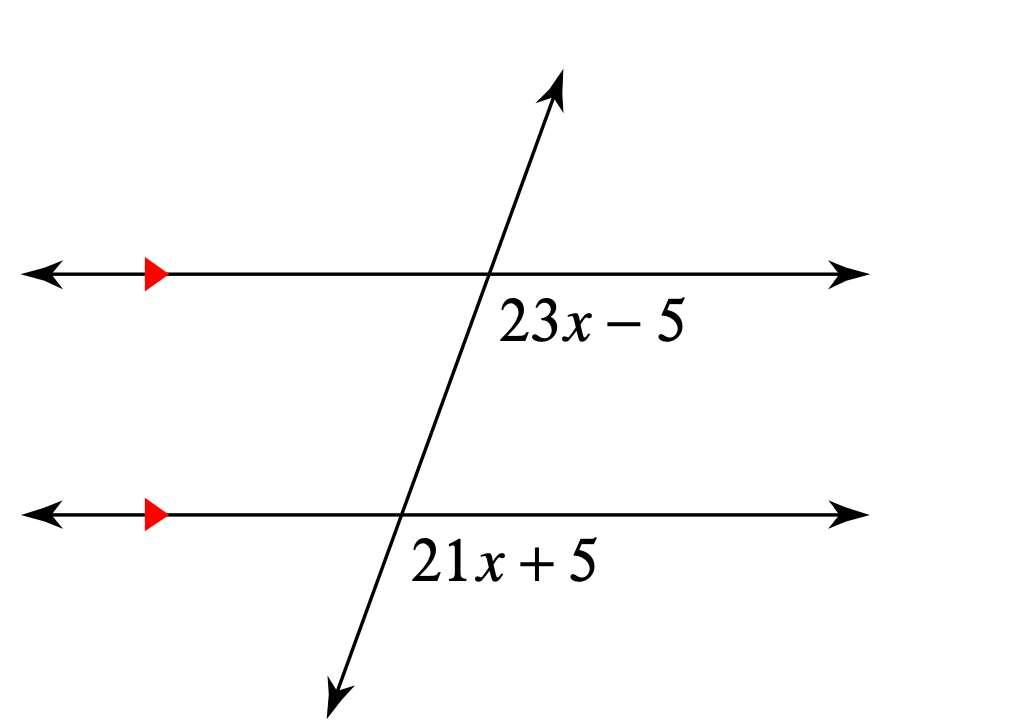x = 5

400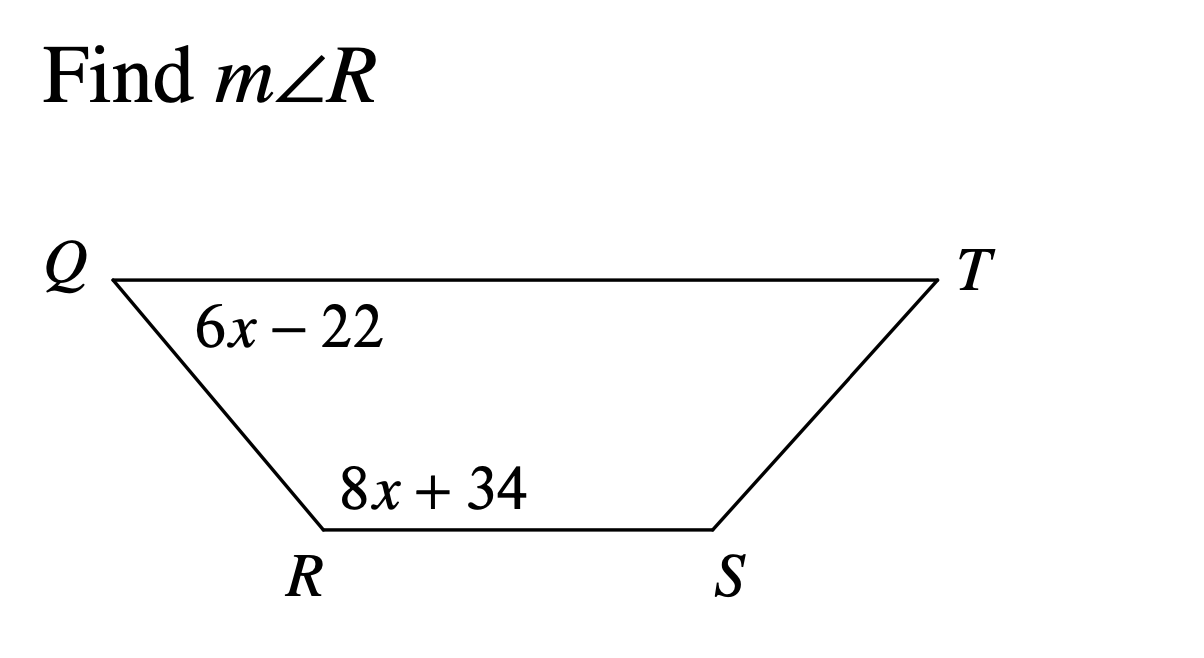m\angle R = 130^o

400

What sports did Isaac play in high school?

Football and Lacrosse

500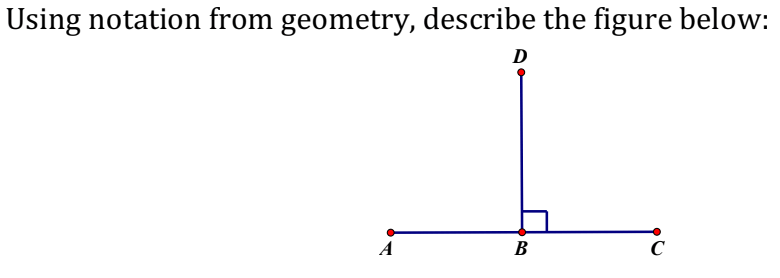\overline{AC} \bot \overline{DB}

500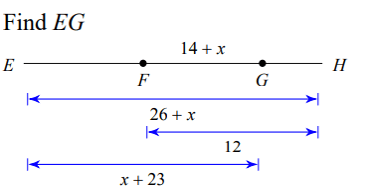EG = 18

500

Find the value of x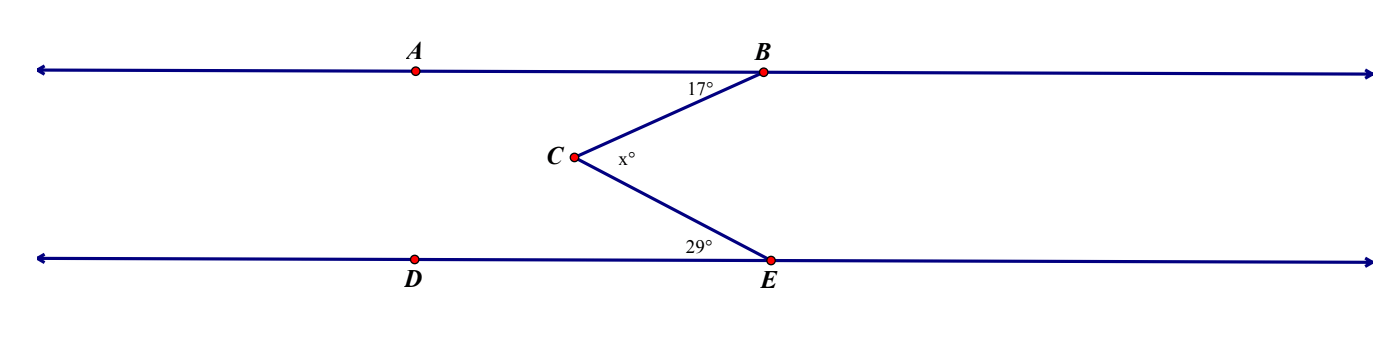x = 46

500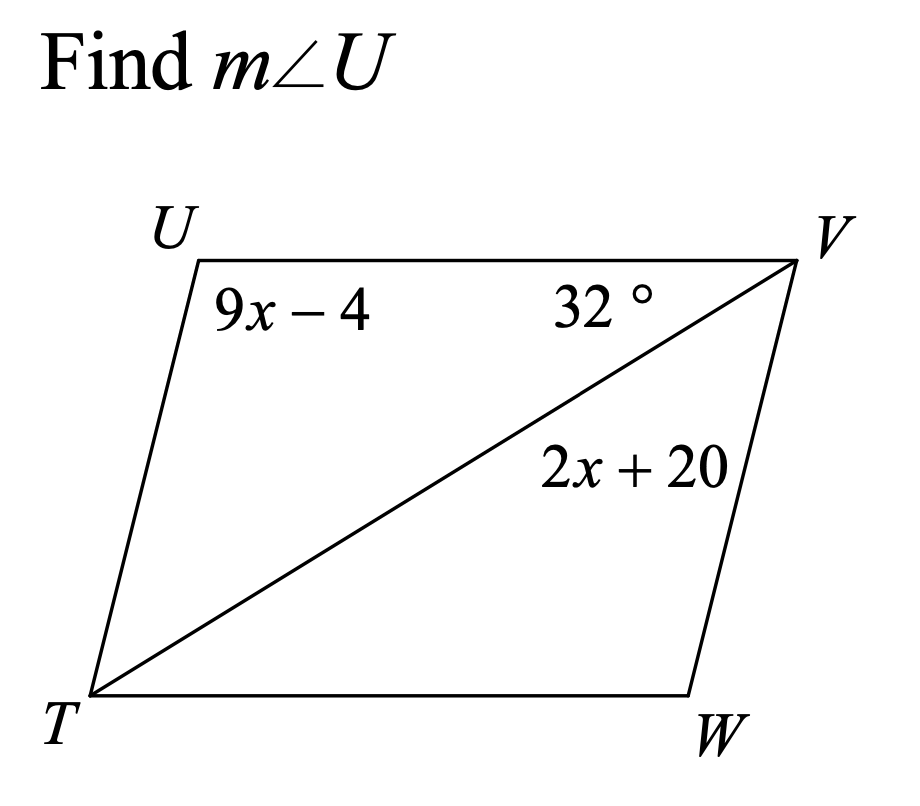m\angle U =104^o

500

Which of the following is NOT a hobby of Isaac's?

a) Writing
b) Climbing
c) Hiking
d) Drawing

Drawing

Click to zoom